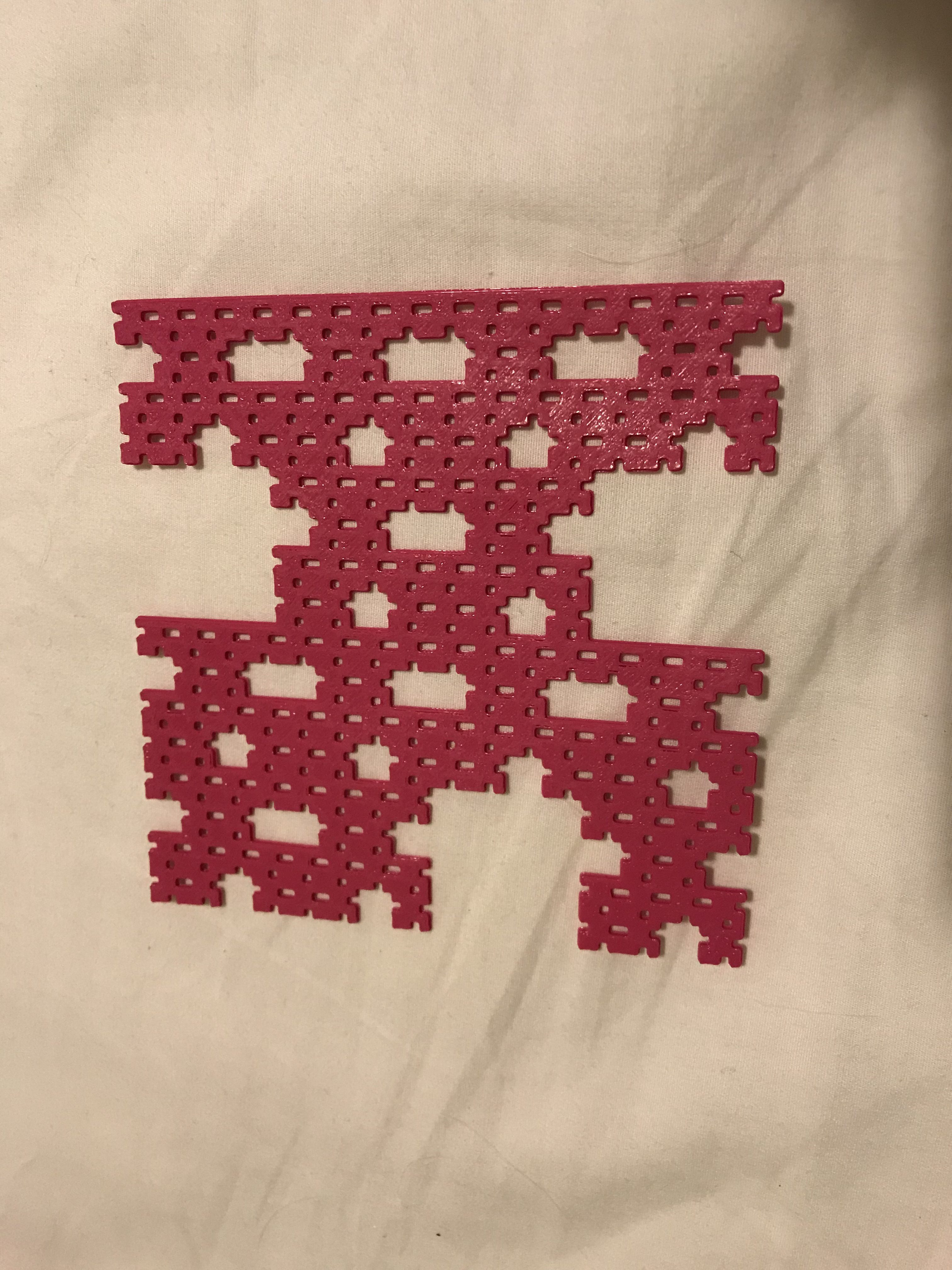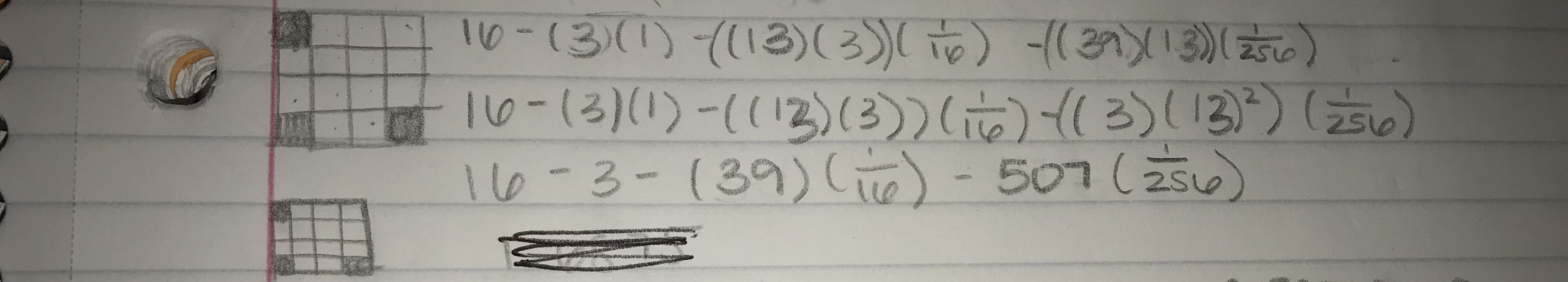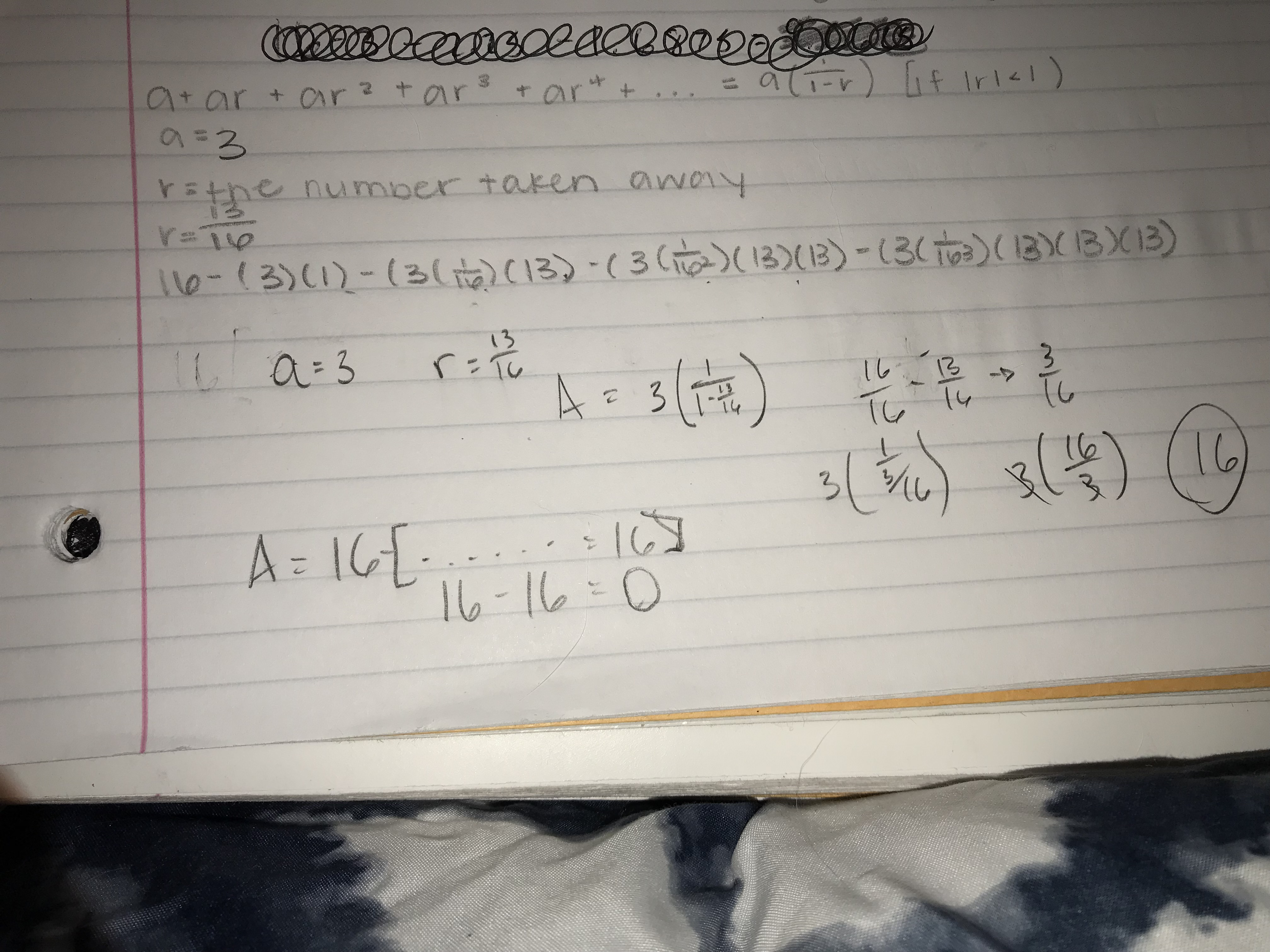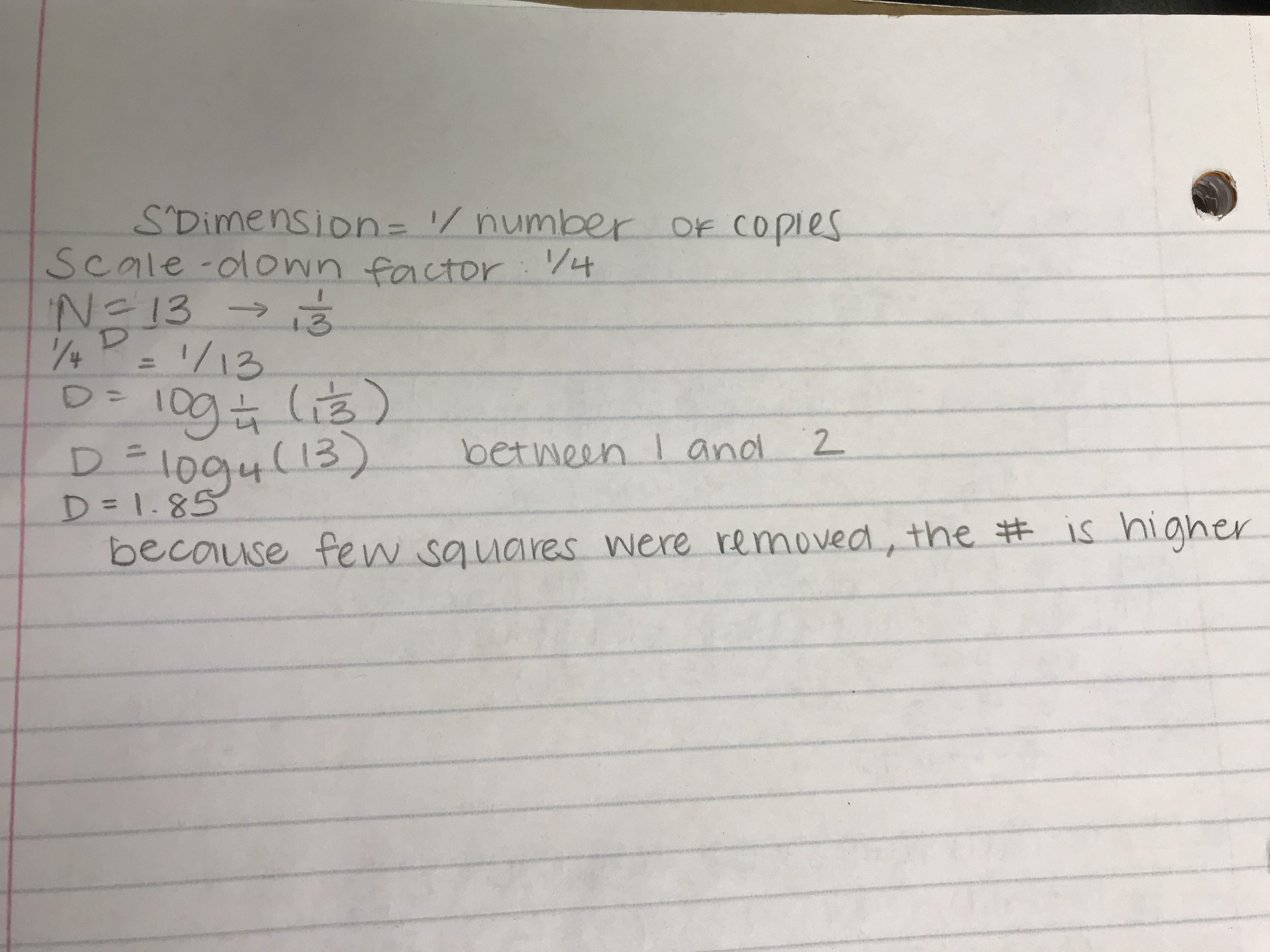# House Fractal

## House Fractal Level 3I named my fractal the House Fractal because I think the removed squares resemble little tiny houses with chimneys on the roofs. To create this, I removed (0,1), (1,3), and (3,1).## Level 1

The surface area for level one would be 13 because we started out with 16 squares total, and I removed 3.

16-(3)(1)=13

## Level 2

The surface area for level 2 would be 2.4375. You continue adding onto the previous equation to find the surface area for the second level. So 13x3x1/16 is the part of the equation reserved for level 2. The whole equation put together looks like this

((13)(3)(1/16))

## Level 3

Finally, my surface area for level 3 is 1.609. Because this is scaled down by a third, your total area is 1/256. In addition, you have to increase the power of your level because you’ve scaled down your fractal twice now. In level four, you would have to increase the surface area of level 1 to 3. Here’s the total equation written out:

((3)(13^2)(1/256))

## Total Surface Area

My total surface area is 8.58263125. That it found by following the entire equation in the picture above.Thanks to a friend in the class helping me, I figured out that my fractal could go on infinitely. The reason for that being  the total surface area cancels out with my math once it’s finished meaning that the total surface area is 0, which is impossible. To explain my math a little bit better, a=3 because that’s the number of squares I removed. R=13/16 because 13 is the surface area of level 1 with the squares removed over the total surface area. R stays the same because every unit of the following levels has 13/16 squares present.

## Dimension

The dimension of a fractal carpet should be between 1 and 2. A regular line would have a dimension of 1, and a square would have a dimension of 2. A fractal isn’t somewhere in between a one-dimensional and two-dimensional object. To calculate the dimension of my fractal, I took the scale-down factor (S) to the power of D and set all of that equal to 1 over the number of copies. Since I took 3 squares out of my original 16, my number of copies was 13. My scale-down factor was 1/4 because one box is a quarter of the whole. The final equation looks like this:

D = log_4(13)

Instead of 1/13 and 1/4, you just flip the numerators and denominators because the denominators have to be equal, so they both are set to 1.My final dimension ended up being around 1.85. My final dimension is on the higher side because I took fewer squares out of my original, so it more closely resembles a complete square.